### 11.4.3.3 Derived I-spaces for continuous time

The continuous-time case is substantially more difficult, both to express and to compute in general forms. In many special cases, however, there are elegant ways to compute it. Some of these will be covered in Section 11.5 and Chapter 12. To help complete the I-space framework, some general expressions are given here. In general, I-maps and derived I-spaces can be constructed following the ideas of Section 11.2.1.

Since there are no discrete transition rules, the derived I-states cannot be expressed in terms of simple update rules. However, they can at least be expressed as a function that indicates the state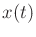that will be obtained after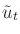and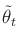are applied from an initial state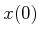. Often, this is obtained via some form of integration (see Section 14.1), although this may not be explicitly given. In general, let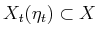denote a nondeterministic I-state at time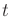; this is the replacement for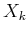from the discrete-stage case. The initial condition is denoted as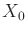, as opposed to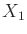, which was used in the discrete-stage case.

More definitions are needed to precisely characterize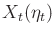. Let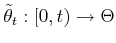denote the history of nature actions up to time. Similarly, let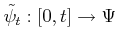denote the history of nature sensing actions. Suppose that the initial condition is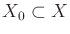. The nondeterministic I-state is defined as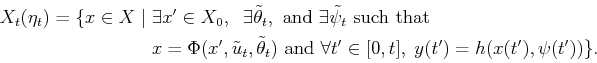(11.66)

In words, this means that a statelies inif and only if there exists an initial state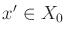, a nature history, and a nature sensing action history,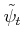such that the transition equation causes arrival atand the observation history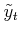agrees with the sensor mapping over all time from 0 to.

It is also possible to derive a probabilistic I-state, but this requires technical details from continuous-time stochastic processes and stochastic differential equations. In some cases, the resulting expressions work out very nicely; however, it is difficult to formulate a general expression for the derived I-state because it depends on many technical assumptions regarding the behavior of the stochastic processes. For details on such systems, see .

Steven M LaValle 2020-08-14